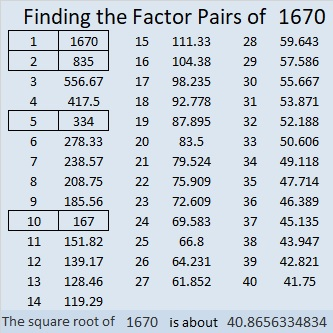# 1670 Mystery Puzzle

Contents

### Today’s Puzzle:

The logic needed to solve this puzzle is a bit complicated. If you would like a hint, I’ll give one later in the post that will remove most of the mystery in the puzzle. Write the numbers 1 to 12 in both the first column and the top row so that those numbers and the given clues become a multiplication table.Logic Hint:
The 33 means that the 9 cannot be 3 × 3, so the 9 and the 54 must use both 9’s.
The 54 also must use a 6.
Thus the 36 near the bottom of the puzzle cannot be 9 × 4 or 6 × 6 and must be 3 × 12.
84 and that bottom 36 must use both 12’s, so the 48 must be 6 × 8 and not 12 × 4.
The rest of the puzzle should be rather straightforward.

### Factors of 1670:

• 1670 is a composite number.
• Prime factorization: 1670 = 2 × 5 × 167.
• 1670 has no exponents greater than 1 in its prime factorization, so √1670 cannot be simplified.
• The exponents in the prime factorization are 1, 1, and 1. Adding one to each exponent and multiplying we get (1 + 1)(1 + 1)(1 + 1) = 2 × 2 × 2 = 8. Therefore 1670 has exactly 8 factors.
• The factors of 1670 are outlined with their factor pair partners in the graphic below.### More About the Number 1670:

1670 is the hypotenuse of a Pythagorean triple:
1002-1336-1670, which is (3-4-5) times 334.

Although it is very much irrational, OEIS.org informs us that the first few digits of
1670^(1/6) is 3.44444624848…

This site uses Akismet to reduce spam. Learn how your comment data is processed.